# Golf Course Optimization with Raspberry Pi and Hologram Nova

Combines the cellular capability of Hologram Nova with the small-package power of Raspberry Pi Zero W to track real-time golfer movements.

IntermediateFull instructions providedOver 1 day2,131## Things used in this project

### Hardware components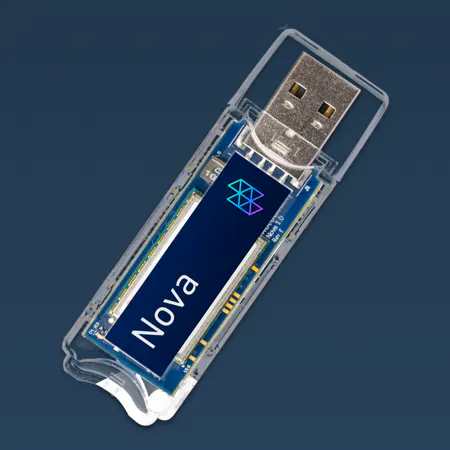Hologram Nova
×1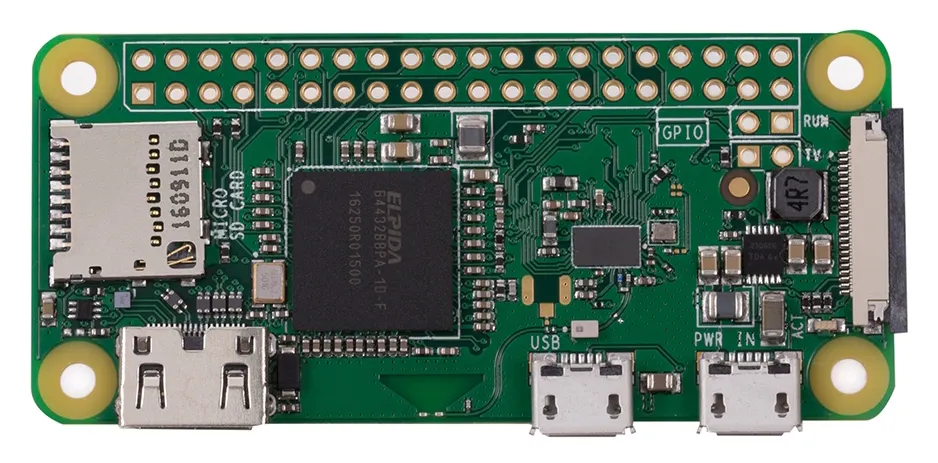Raspberry Pi Zero Wireless
×1
×1
 External Battery
×1
 USB Hub
×1
 Waterproof project box
×1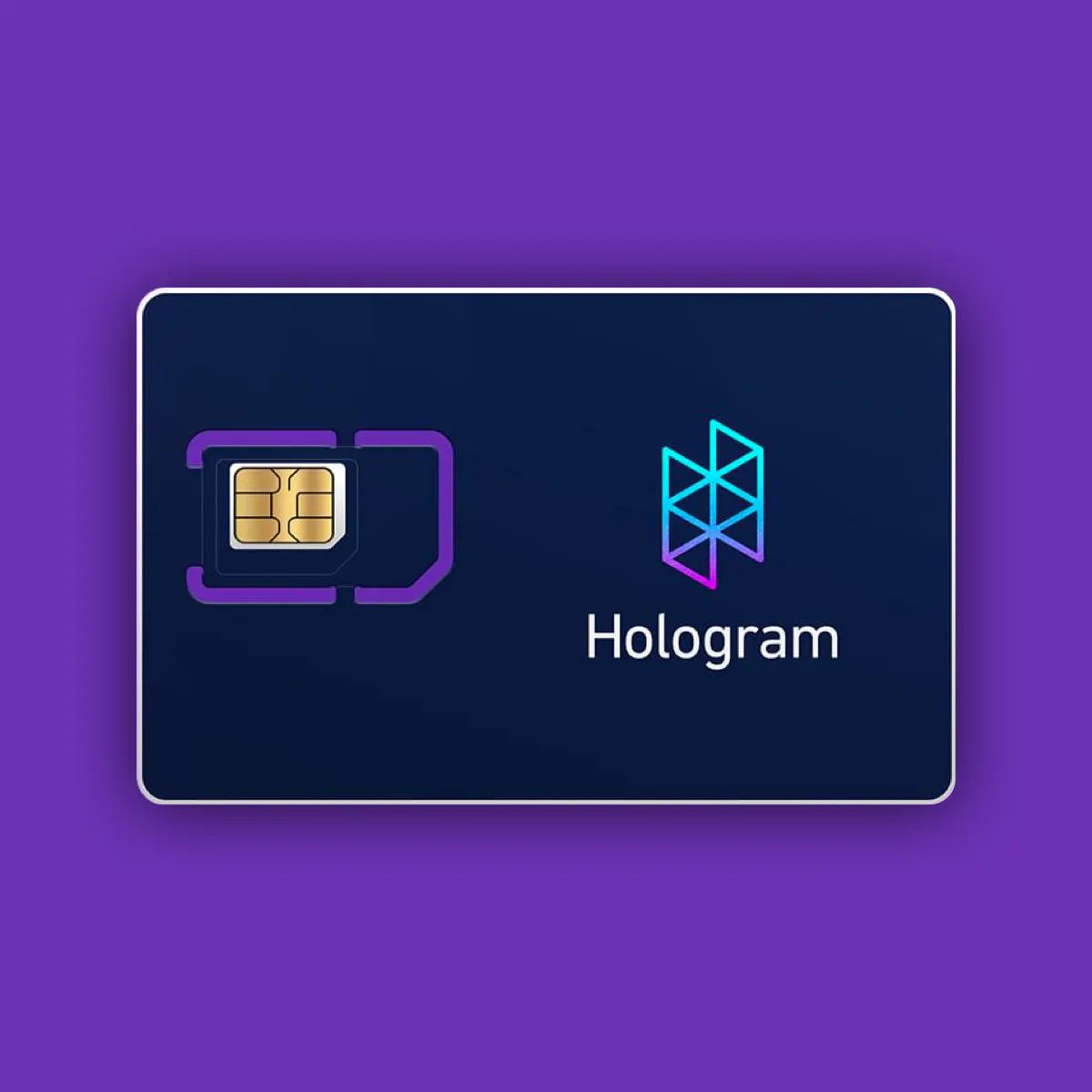Hologram Global IoT SIM Card
×1

### Software apps and online services

 gps.py Matt Richardson's basic Python code for GPS data processing, featured in Magpi issue 40
 Hologram Python SDK
 ThingsBoard.io

### Hand tools and fabrication machines

 Bottle opener
 Anchor Steam

## Schematics

### Golfer Tracker diagram

Real-time golfer position data is tracked from GPS to RPi Zero W, transmitted to Google Sheet via Hologram Nova, and visualized for course management on Google Earth.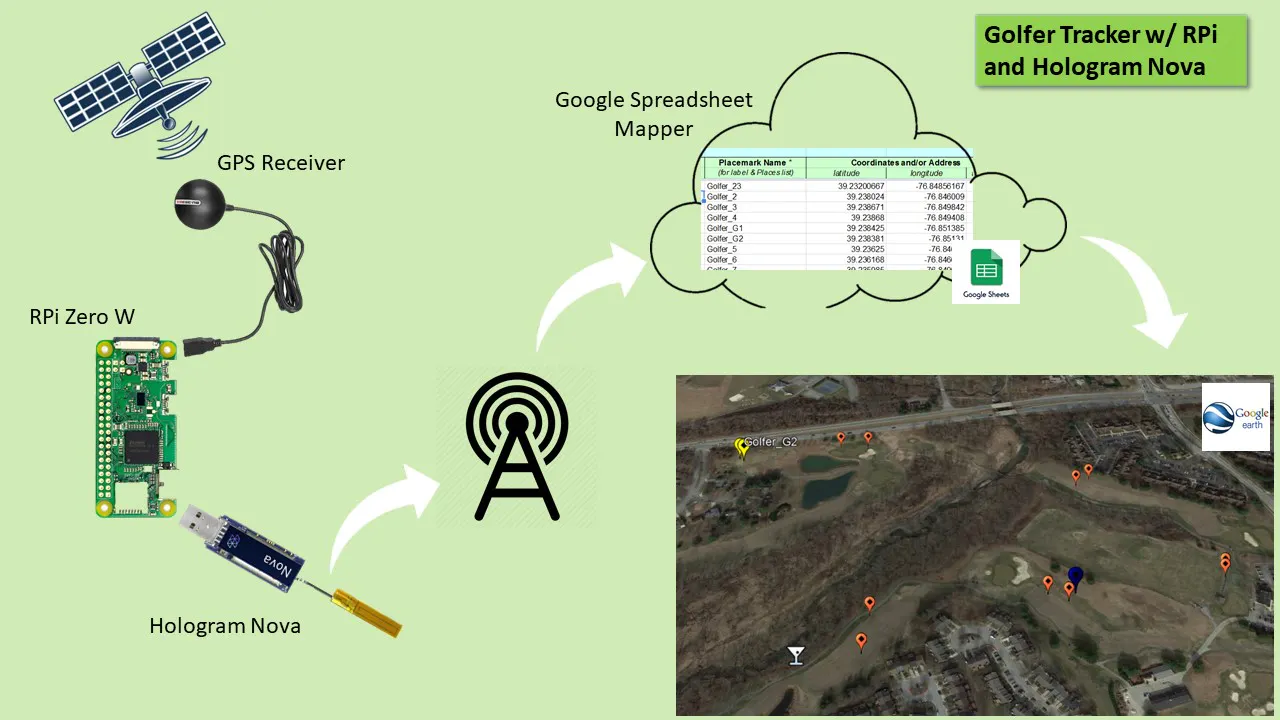### Golfer Tracker diagram v2

Version 2 replaces Google Sheets/Earth with ThingsBoard.io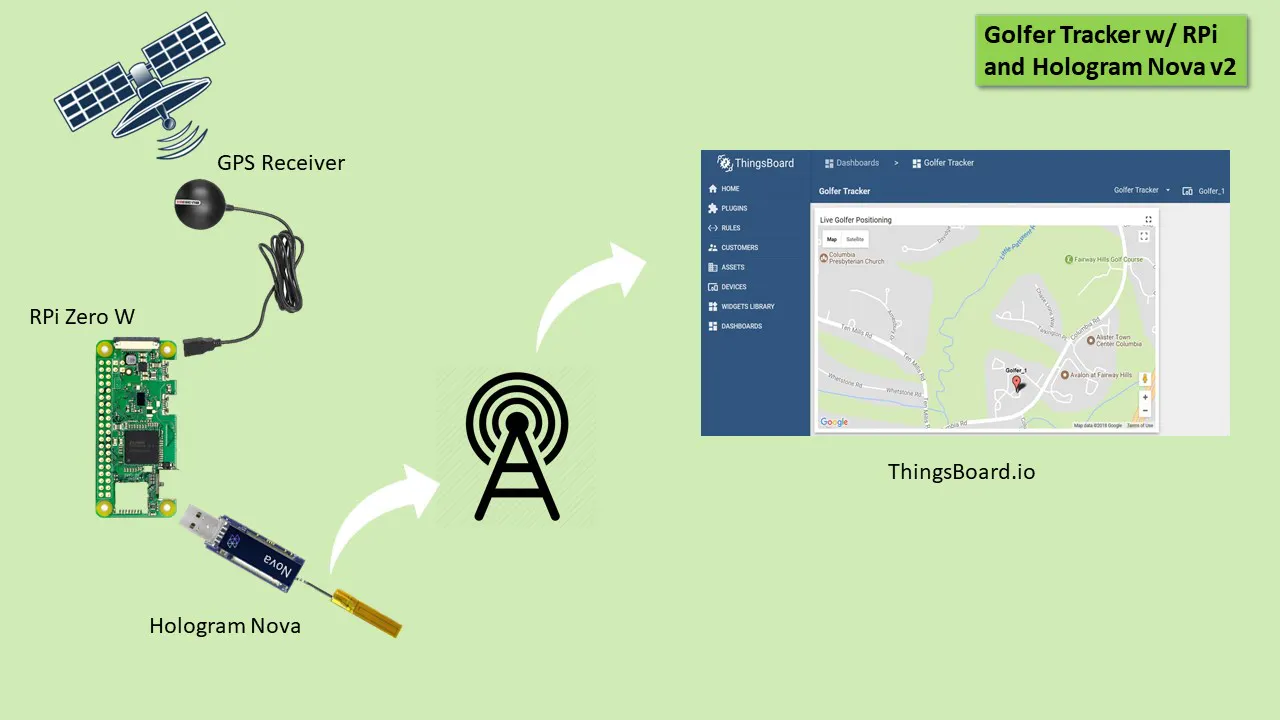## Code

### Golfer GPS Tracking

Python
Core components are:
b) Hologram Python SDK for managing cellular communications
d) GPS data processing
e) Log file of the extended golf outing path
```import serial
import os

# Library for managing Google Sheets update and authentication

from oauth2client.service_account import ServiceAccountCredentials

# Hologram cloud library for cellular comminucations
from Hologram.HologramCloud import HologramCloud

hologram = HologramCloud(dict(), network='cellular')

result = hologram.network.connect()
if result == False:
print ('Failed to connect to cell network')

# Use creds to create a client to interact with the Google Drive API
creds = ServiceAccountCredentials.from_json_keyfile_name('client_secret.json', scope)

# Name of the Google Sheet and the specific worksheet
sheet = client.open("Golfer Tracker").worksheet("PlacemarkData")

firstFixFlag = False # this will go true after the first GPS fix.
firstFixDate = ""

# Set up serial:
ser = serial.Serial(
port='/dev/ttyUSB0',\
baudrate=4800,\
parity=serial.PARITY_NONE,\
stopbits=serial.STOPBITS_ONE,\
bytesize=serial.EIGHTBITS,\
timeout=1)

# Helper function to take HHMM.SS, Hemisphere and make it decimal:
def degrees_to_decimal(data, hemisphere):
try:
decimalPointPosition = data.index('.')
degrees = float(data[:decimalPointPosition-2])
minutes = float(data[decimalPointPosition-2:])/60
output = degrees + minutes
if hemisphere is 'N' or hemisphere is 'E':
return output
if hemisphere is 'S' or hemisphere is 'W':
return -output
except:
return ""

# Helper function to take a \$GPRMC sentence, and turn it into a Python dictionary.
# This also calls degrees_to_decimal and stores the decimal values as well.
def parse_GPRMC(data):
data = data.split(',')
dict = {
'fix_time': data,
'validity': data,
'latitude': data,
'latitude_hemisphere' : data,
'longitude' : data,
'longitude_hemisphere' : data,
'speed': data,
'true_course': data,
'fix_date': data,
'variation': data,
'variation_e_w' : data,
'checksum' : data
}
dict['decimal_latitude'] = degrees_to_decimal(dict['latitude'], dict['latitude_hemisphere'])
dict['decimal_longitude'] = degrees_to_decimal(dict['longitude'], dict['longitude_hemisphere'])
return dict

# Main program loop:
while True:
if "\$GPRMC" in line: # This will exclude other NMEA sentences the GPS unit provides
gpsData = parse_GPRMC(line) # Turn a GPRMC sentence into a Python dictionary called gpsData
if gpsData['validity'] == "A": # If the sentence shows that there's a fix, then we can log the line
if firstFixFlag is False: # If we haven't found a fix before, then set the filename prefix with GPS date & time.
firstFixDate = gpsData['fix_date'] + "-" + gpsData['fix_time']
firstFixFlag = True
else: # write the data to a simple log file and then to Google Sheets:
with open("/home/pi/gps_experimentation/" + firstFixDate +"-simple-log.txt", "a") as myfile:
myfile.write(gpsData['fix_date'] + "," + gpsData['fix_time'] + "," + str(gpsData['decimal_latitude']) + "," + str(gpsData['decimal_longitude']) +"\n")
print (gpsData['fix_date'] + "," + gpsData['fix_time'] + "," + str(gpsData['decimal_latitude']) + "," + str(gpsData['decimal_longitude']) +"\n")
sheet.update_cell(11, 5, str(gpsData['decimal_latitude'])) # Write location data to the Google Sheet
sheet.update_cell(11, 6, str(gpsData['decimal_longitude']))
# Disconnect from the cellular network
hologram.network.disconnect()

```

### Golfer GPS Tracking v2

Python
Two changes:
1) Interval timer added to reduce cellular data usage
2) Simplified course load visualization using MQTT and provided dashboards on Thingsboard.io

(See "Version 2" change comments in the code below)
```import serial
import os

# Library for managing Google Sheets update and authentication

# Version 2 libraries:
import time
import sys
import paho.mqtt.client as mqtt
import json

from oauth2client.service_account import ServiceAccountCredentials

# Hologram cloud library for cellular comminucations
from Hologram.HologramCloud import HologramCloud

hologram = HologramCloud(dict(), network='cellular')

result = hologram.network.connect()
if result == False:
print ('Failed to connect to cell network')

# Use creds to create a client to interact with the Google Drive API
creds = ServiceAccountCredentials.from_json_keyfile_name('client_secret.json', scope)

# Name of the Google Sheet and the specific worksheet
sheet = client.open("Golfer Tracker").worksheet("PlacemarkData")

THINGSBOARD_HOST = 'demo.thingsboard.io'

# Version 2 - Specify interval to reduce cellular data usage
INTERVAL=10

# Version 2 - Initialize mqtt
gps_data = {'latitude': 0, 'longitude': 0}
client = mqtt.Client()

# Version 2 - Connect to ThingsBoard using default MQTT port and 60 seconds keepalive interval
client.connect(THINGSBOARD_HOST, 1883, 60)
client.loop_start()

# GPS fix flag will go true after first fix
firstFixFlag = False
firstFixDate = ""

# Set up serial:
ser = serial.Serial(
port='/dev/ttyUSB0',\
baudrate=4800,\
parity=serial.PARITY_NONE,\
stopbits=serial.STOPBITS_ONE,\
bytesize=serial.EIGHTBITS,\
timeout=1)

# Helper function to take HHMM.SS, Hemisphere and make it decimal:
def degrees_to_decimal(data, hemisphere):
try:
decimalPointPosition = data.index('.')
degrees = float(data[:decimalPointPosition-2])
minutes = float(data[decimalPointPosition-2:])/60
output = degrees + minutes
if hemisphere is 'N' or hemisphere is 'E':
return output
if hemisphere is 'S' or hemisphere is 'W':
return -output
except:
return ""

# Helper function to take a \$GPRMC sentence, and turn it into a Python dictionary.
# This also calls degrees_to_decimal and stores the decimal values as well.
def parse_GPRMC(data):
data = data.split(',')
dict = {
'fix_time': data,
'validity': data,
'latitude': data,
'latitude_hemisphere' : data,
'longitude' : data,
'longitude_hemisphere' : data,
'speed': data,
'true_course': data,
'fix_date': data,
'variation': data,
'variation_e_w' : data,
'checksum' : data
}
dict['decimal_latitude'] = degrees_to_decimal(dict['latitude'], dict['latitude_hemisphere'])
dict['decimal_longitude'] = degrees_to_decimal(dict['longitude'], dict['longitude_hemisphere'])
return dict

# Main program loop:
while True:
if "\$GPRMC" in line: # This will exclude other NMEA sentences the GPS unit provides
gpsData = parse_GPRMC(line) # Turn a GPRMC sentence into a Python dictionary called gpsData
if gpsData['validity'] == "A": # If the sentence shows that there's a fix, then we can log the line
if firstFixFlag is False: # If we haven't found a fix before, then set the filename prefix with GPS date & time.
firstFixDate = gpsData['fix_date'] + "-" + gpsData['fix_time']
firstFixFlag = True
else: # write the data to a simple log file and then to Google Sheets:
with open("/home/pi/gps_experimentation/" + firstFixDate +"-simple-log.txt", "a") as myfile:
myfile.write(gpsData['fix_date'] + "," + gpsData['fix_time'] + "," + str(gpsData['decimal_latitude']) + "," + str(gpsData['decimal_longitude']) +"\n")
print (gpsData['fix_date'] + "," + gpsData['fix_time'] + "," + str(gpsData['decimal_latitude']) + "," + str(gpsData['decimal_longitude']) +"\n")
# Write location data to the Google Sheet
sheet.update_cell(11, 5, str(gpsData['decimal_latitude']))
sheet.update_cell(11, 6, str(gpsData['decimal_longitude']))
# Version 2 - Write location data to Thingsboard
gps_data['latitude'] = str(gpsData['decimal_latitude'])
gps_data['longitude'] = str(gpsData['decimal_longitude'])
client.publish('v1/devices/me/telemetry', json.dumps(gps_data), 1)

# Version 2 - Interval timing control
if sleep_time > 0:
time.sleep(sleep_time)

client.loop_stop()
client.disconnect()

# Disconnect from the cellular network
hologram.network.disconnect()

```

## Credits

### tnunnster

1 project • 4 followers
Little Lebowski Urban Achiever and part-time Abider. Very appreciative of the community and the open network of project ideas.